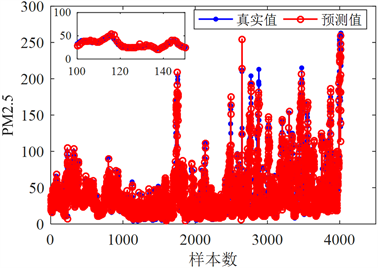﻿ 一种基于随机配置网络的PM2.5浓度预测方法

# 一种基于随机配置网络的PM2.5浓度预测方法A Method of Predicting PM2.5 Using Stochastic Configuration Network

Abstract: As a key index of measuring the air quality, fine particulate matter (PM2.5) plays an important role in realizing the environmental treatment. The air quality can be effectively improved by reducing the PM2.5 concentration, which can prevent the occurrence of various kinds of respiratory diseases. Hence, it is very important to predict the PM2.5. In this paper, using the stochastic configuration network algorithm, a nonlinear regression model is established to predict the PM2.5. Experiment result illustrates that the established PM2.5 prediction model using the stochastic configuration network has a high precision.

1. 引言

2. 预备知识

${〈{e}_{L-1},{g}_{L}〉}^{2}\ge {g}_{L}^{2}\left(1-r-{u}_{L}\right){‖{e}_{L-1}‖}^{2}$ (1)

$\left[{\beta }_{1}^{*},{\beta }_{2}^{*},\cdots ,{\beta }_{L}^{*}\right]=\mathrm{arg}\underset{\beta }{\mathrm{min}}‖f-\underset{j=1}{\overset{L}{\sum }}{\beta }_{j}{g}_{j}‖$ (2)

3. 基于随机配置网络的PM2.5浓度预测模型

3.1. 数据预处理

for i=size(Xtraino, 1):-1:1

if sum(isnan(Xtraino(i, :))) > 0

Xtraino(i, :)=[];

end

end

delete(' Xtrain.csv');

dlmwrite('Xtrain.csv', Xtraino, 'precision', 12);Table 1. Some original training data

Table 2. The corrected training data

3.2. 数据的归一化

[Xntrain,inputps]= mapminmax(Xtrain);

Xntest=mapminmax('apply',Xtest,inputps);

[Yntrain,outputps]=mapminmax(Ytrain);

Yntest=mapminmax('apply',Ytest,outputps);

3.3. 训练集与测试集

3.4. PM2.5浓度预测模型的实现

$X=\left\{{x}_{1},{x}_{2},\cdots ,{x}_{8218}\right\},{x}_{i}=\left\{{x}_{i1},{x}_{i2},\cdots ,{x}_{i12}\right\}\in {R}^{12}$ 为训练模型的输入，则对应的模型的输出为 $Y=\left\{{y}_{1},{y}_{2},\cdots ,{y}_{8218}\right\},{y}_{i}=\left\{{y}_{i1}\right\}\in {R}^{1}$。给定两个空集 $\Omega$ 和W，用于存储监督机制所产生的隐层参数w和b。模型的具体实现步骤如下：

1) 隐含层节点的增加。首先在监督机制下随机配置一组隐层参数，若找不到满足监督机制的节点，则对监督机制的约束力度进行放松，即 $r=r+\tau$$\tau \in \left(0,1-r\right)$，直至找到监督机制下的隐层参数为止。将获得的隐性参数存储于 $\Omega$ 和W中，再从中找出一组隐性参数，即 ${w}^{*}$${b}^{*}$，使得当前模型具有最高的预测精度，并将其代入事先指定的激活函数，形成新增节点。

2) 输出权值的更新。在确定激活函数的随机参数后，需要对隐含层与输出层之间的连接权值进行更新。本文采用全局优化算法(2)来得到网络的输出权值。最后，重复步骤1)和2)，直至到达设置的停止条件，模型建立完毕。

3.5. 模型参数选择

${L}_{\mathrm{max}}=100$${T}_{\mathrm{max}}=200$$r=0.9$$\epsilon =0.01$$\Upsilon :=\left\{1:1:20\right\}$

4. 模型验证Figure 1. Prediction result of the PM2.5 based on stochastic configuration network

5. 结论

 刘基伟, 闵素芹, 金梦迪. 基于分布式感知深度神经网络的高分辨率PM2.5值估算[J]. 地理学报, 2021, 76(1): 191-205.

 王磊, 杨翠丽, 乔俊飞. 基于回声状态网络的PM2.5预测研究[J]. 控制工程, 2019, 26(1): 1-5.

 段大高, 赵振东, 梁少虎, 等. 基于LSTM的PM2.5浓度预测模型[J]. 计算机测量与控制, 2019, 27(3): 215-219.

 郭云, 李瑞娟, 黄炳昭, 等. 未来10年保定市大气PM2.5的健康效益预测[J]. 中国环境科学, 2020, 40(12): 5459-5467.

 米馨, 张云婷, 胡立文, 等. 大气PM2.5长期暴露与儿童血压升高的关联研究[J]. 中华预防医学杂志, 2019, 53(1): 45-50.

 代伟, 李德鹏, 杨春雨, 等. 一种随机配置网络的模型与数据混合并行学习方法[J]. 自动化学报, 2019(45): 1-11.

 张洁, 庞丽萍, 曲洪权, 等. 基于随机配置网络的机载电子吊舱多工况热模型[J]. 化工学报, 2020, 71(S1): 441-447.

 张万栋, 李庆忠, 黎明, 等. 基于最优误差自校正极限学习机的高频地波雷达RD谱图海面目标检测算法[J]. 自动化学报, 2021, 47(1): 108-120.

 夏平凡, 倪志伟, 朱旭辉, 等. 基于双错测度的极限学习机选择性集成方法[J]. 电子与信息学报, 2020, 42(11): 2756-2764.

Top